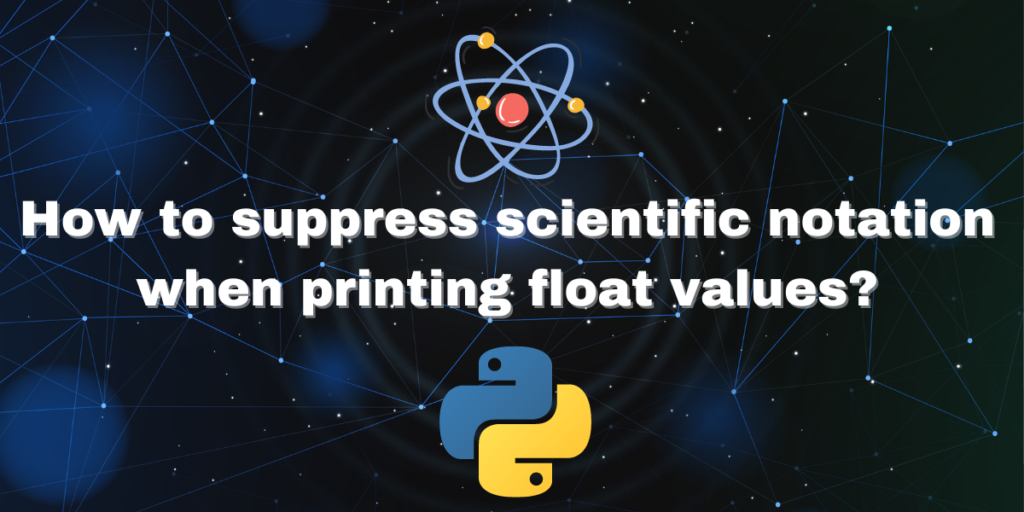# Suppressing Scientific Notation in Python for Float ValuesScientific notation is a form of expression used to represent very large numbers or extremely small numbers in mathematics. It is the simpler form of those numbers that are easier to read and comprehend. Numbers such as irrational numbers or whole numbers, can be expanded up to millions of places after the decimal point.

Sometimes we don’t need the entirety of those numbers printed on the output screen of a program, making it messy and hard to read. Hence, scientific notation can be used to save space and make the output look nice and crispy. But if you want to display the entire number, there are ways to do it in Python.

Scientific notation can be represented in a general form and there are some rules to it. We’ll take a look at all of those in this tutorial and also go through the function in Python that will help us format tiny or large numbers according to our use case.

## General Form and Rules of Scientific Notation

The general form of a number represented in scientific notation looks something like this:

```X * 10y
```

In the above expression, X is the mantissa and 10y is the exponent. The exponent is used to determine the place of the decimal point and the mantissa is used for representing the main digits of the number in base 10.

There are some rules to using scientific notations. They are:

• The mantissa will always represent the main digits of the decimal number.
• The number should always be represented in base 10, that is, it must always be a decimal number.
• The value of y in 10y cannot be zero, y>0 or y<0.
• The absolute value of X would be between 1 to 10 but not more than 10 (1<X<10).
• The mantissa can be positive or negative.

## Examples of Decimal Numbers in Scientific Notation

Example 1:

The number 68000000000000000 can be represented as 6.8 * 1016 in scientific notation. Here, the exponent is 16 which is positive. The value of the mantissa in this expression lies between 1 and 10.

Example 2:

The number 0.0000000081 can be represented as 8.1 * 10-9 in scientific notation. Here, the exponent is -9 which is negative. The value of mantissa is also lying between 1 and 10.

## Using Scientific Notation in Python

In this section, we will look at a program that will display the results in the scientific notation in Python. The power of 10, that is the exponent portion of the scientific notation in programming languages is represented as “ez” where ‘e’ represents “exponent” and “z” is the power.

Do check out: Python Built-in Functions: Brief Overview.

## Suppressing Scientific Notation in Python

In Python, you can suppress scientific notation when printing float values by using the format function. The syntax to suppress scientific notation is f'{number:.(required_number_of_decimal_places)f}’. For example, to display 9 decimal places, use f'{result:.9f}’. This will display the entire number, even if it’s very large or very small, instead of using scientific notation.

The format function in python can be used to suppress the display of scientific notation. The syntax of the function is as shown below:

```f'{number:.(required_number_of_decimal_places)f}'
```

For example, if you only need 9 points after the decimal point, it may be written as `f'{result:.9f}'`

Let’s look at an example:

```#code for showing suppressing the representation of scientific notation in Python
a= 14.0
b = 20000000.0
result= a/b
#formatting the result
result=f'{result:.9f}'
print("The result is=",result)
```

Similar: Understanding the Print Format %.3f in Python.

## Disadvantages of Suppressing Scientific Notation

There are also some disadvantages of suppressing the scientific notation in Python. Some of them are:

• It takes up more space and the variable, if extremely large, might slow down the speed of the program as a whole.
• In extremely big scientific calculations, suppressing scientific notation might cause errors.
• The output displayed may become hard to read.